Publication date: 08/13/2020

## Tests Report

#### Rsquare (U)

Rsquare (U) is computed as follows: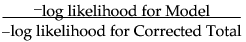The total negative log-likelihood is found by fitting fixed response rates across the total sample.

#### Test

The two Chi-square tests are as follows:

The Likelihood Ratio Chi-square test statistic is computed as twice the negative log-likelihood for Model in the Tests table. Some books use the notation G2 for this statistic. The difference of two negative log-likelihoods, one with whole-population response probabilities and one with each-population response rates, is written as follows: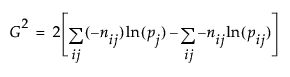where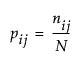and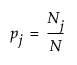This formula can be more compactly written as follows: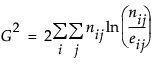The Pearson Chi-square test statistic is calculated by summing the squares of the differences between the observed and expected cell counts. The Pearson Chi-square test exploits the property that frequency counts tend to a normal distribution in very large samples. The familiar form of this Chi-square statistic is as follows: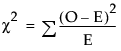where O is the observed cell counts and E is the expected cell counts. The summation is over all cells. There is no continuity correction done here, as is sometimes done in 2-by-2 tables.